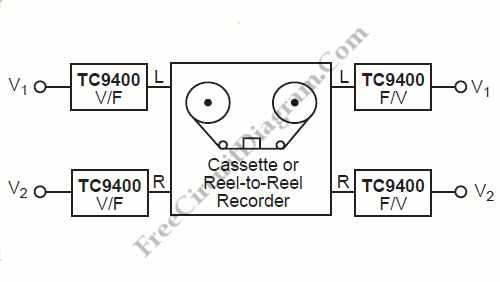Interfacing High Power Load to Logic Level with Optocoupler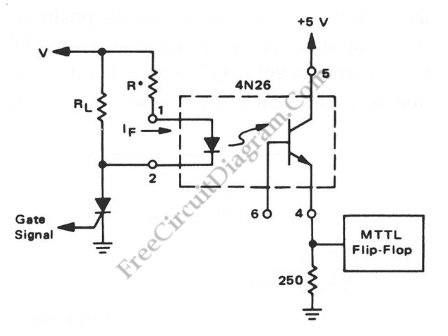Interfacing high power load can be done in many ways, such as using voltage divider resistors, transformer, or optocoupler.  In the circuit shown below , an optocoupler method is presented. This optocoupler serve the logic level translation with flexible input, accepting wide range of voltage level, which is suitable for many high power load/devices. To couple a high-voltage load to […]

Three Wire RS232 To RS485 Converter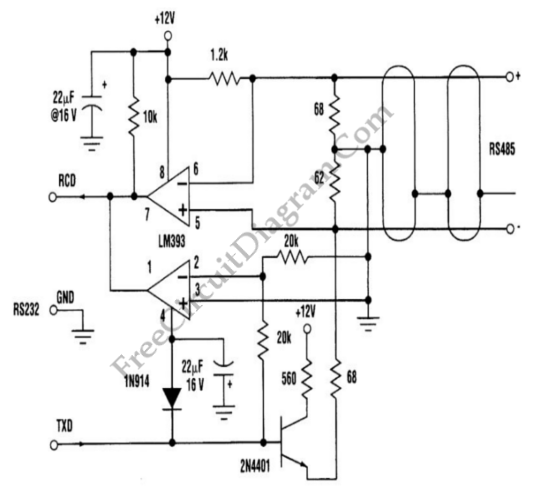The schematic diagram below show the circuit diagram of an RS232 to RS485 converter circuit. The  EIA-485 standard, a.k.a TIA/EIA-485 or RS-485, is a physical layer standard defining the electrical properties of electronic drivers and receivers using balanced digital signal which is capable of handling multipoint systems. The ANSI Telecommunications Industry Association/Electronic Industries Alliance (TIA/EIA) is the organization who publish […]

30-Khz Bandwidth High Linearity Opto-Isolated Amplifier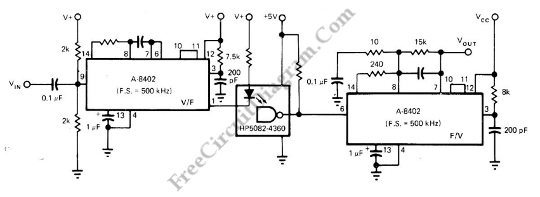Using optocoupler to directly transfer a linear (analog) voltage would produce  a large unpredictable linearity error, which is also sensitive to temperature changes. By using frequency modulation, the total system’s linearity will  depend only on the linearity of the V/F and F/V converter, which is fortunately cancel each other if we use similar devices with similar non-linearity error characteristic. A […]

Pulse Width to Analog Voltage Demodulator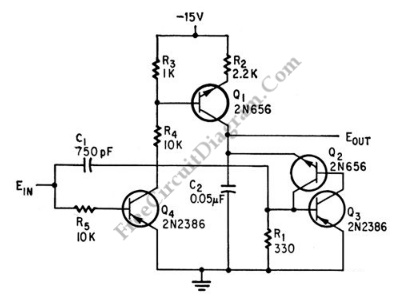This is a schematic diagram of a pulse width to analog demodulator circuit. This circuit is used to demodulate the pulse width to analog voltage level. With input pulse width range of 0 to 1 microsecond, this circuit can produces Output range of 0 to 10 v. The input pulse is integrated and the final value is held until next […]

Superlinear FM (Frequency Modulator)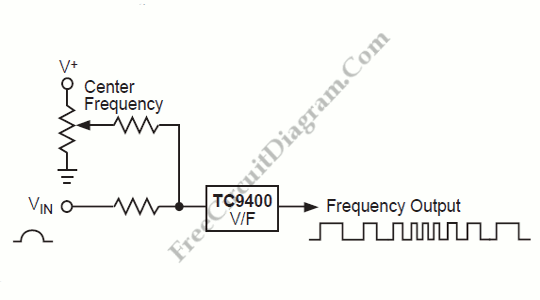Designing various electronic circuit system (synthesizer, modem, decoder, data converter, etc) of ten need a frequency modulator subsystem. An FM modulator is very easy to build with TC9400 since the it has very good linearity as V/F converter. Here is the system’s block diagram: While Vin determines the amount of modulation (FM deviation) around the center frequency, the potentiometer determines […]

Frequency Shift Keying (FSK) Encoding and Decoding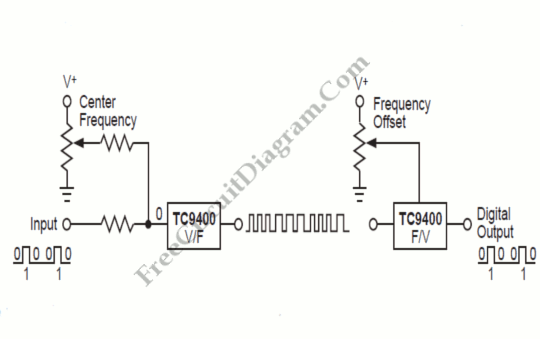The meaning of Frequency Shift Keying (FSK) is transmitting digital data over a signal path (two wires, telephone lines, AM or FM transmitter). Typically, there are only two signals transmitted. One corresponds to a logical “1”. the other to a logical “1”. These two frequency will be generated by a TC9400 V/F converter when connected as shown below. The V/F […]